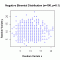I have forgotten

•http://facebook.com/
•https://www.google.com/accounts/o8/id
•https://me.yahoo.com

# Negativebinomial

#### PDF

Calculates the negative binomial distribution PDF.
 double PDF (int x, int r, double p)

#### CDF

Evaluates the negative binomial distribution CDF.
 double CDF (int k, int n, double p, bool upper = false)

#### CDF Inv

The negative binomial distribution inverse CDF
 double CDF_inv (int k, int n, double p, bool upper = false)

#### Random Sample

Generates random numbers following a negative binomial distribution.
 RandomSample::RandomSample unknown (double NX, double PX, double s) RandomSample::~RandomSample unknown () double RandomSample::genReal () double RandomSample::Density (double x)